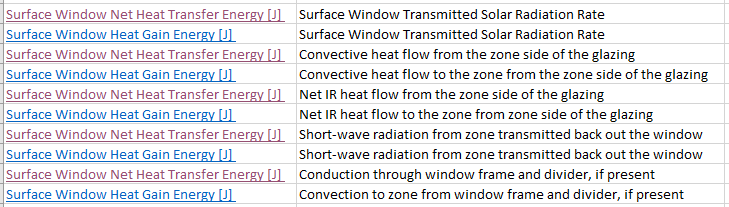Question-and-Answer Resource for the Building Energy Modeling Community
Get started with the Help page

what is the difference betwee "Zone Windows Total Heat Gain Energy" and "Surface Window Heat Gain Energy"?My I ask what the difference between Zone Windows Total Heat Gain Energy and Surface Window Heat Gain Energy is?

It's explained in the E+ document that:

The sum of the heat flow from all of the exterior windows in a zone when that sum is positive.

whereas

Surface Window Heat Gain Energy [J]

The total heat flow to the zone from the glazing, frame and divider of an exterior window when the total heat flow is positive.

Does this mean that the sum of the Surface Window Heat Gain Energy for all the windows of a given zone equals to the Zone Windows Total Heat Gain Energy of that zone?

However, the total across all the zones and surfaces for both variables are not the same, it seems.

edit retag close merge delete

Sort by » oldest newest most voted

You are correct that the "Zone Windows" output is the sum for all "Surface Window" outputs for a given zone. One caveat is that these are both reported "... when that sum is positive" for zone windows and "... when the total heat flow is positive" for individual surface windows ie. when heat is flowing from the exterior to the zone. You may need to also report Surface Window Heat Loss Energy as an output to see when window surfaces have heat flowing from the zone to the exterior. For a given timestep, the sum of all "Surface Window Heat Gain Energy" MINUS "Surface Window Heat Loss Energy" outputs should equal the "Zone Windows Total Heat Gain Energy". Likewise, the sum of all "Surface Window Heat Loss Energy" MINUS "Surface Window Heat Gain Energy" outputs should equal the "Zone Windows Total Heat Loss Energy" output.

Another output to consider is Surface Window Net Heat Transfer Energy, which accounts for radiation from the opaque frame and divider components.

You can read more discussion about window surface outputs on this UnmetHours post.

more

Thank you, Aaron.

I compared the components of both Surface Window Net Heat Transfer Energy and Surface Window Heat Gain/Loss Energy as shown below.

So, is it correct to say that:

...Surface Window Net Heat Transfer Energy is about the net heat flow going through a window surface "itself" (including it's glazing, frame and dividers) from both the outside and inside of the surface through radiation, convection and conduction.

...whereas Surface Window Heat Gain/Loss Energy concerns the "thermal impact" of the zone-side of a window to the zone, i.e., once the window is heated up, how much heat is added to or lost from the zone through the window by means of convection and radiation (no conduction in this case).more

Correct, you summed that up well.

FYI - this should probably be a comment and not a new answer, but I'll let it slide since your table image is helpful for the discussion and wouldn't easily be part of a comment.

Hello, I think energy plus has problem on calculating correct surface window heat transfer. Your table is according to what is written in energy plus reference. In modeling, the result for heat loss and heat gain are not match with energy plus reference description. As I mentioned in my new post:

https://unmethours.com/question/53163...